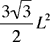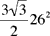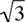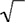Quandaries and Queries Hello.  I need to find the Area in sq. ft. of a Hexagon with 6 equal sides, of 26' each.                                                                       _ I do not understand  the odd (to me) symbol   \/ 3                                                                                   Thank you.                                                                                                       RB Hi RB, I am guessing that you found the area of a regular hexagon to bewhere L is the length of a side. In your case L = 26 feet so te area isThe numberis called the square root of 3. It is the number whose square is 3. There is probably a button on your calculator with the symbolon it. Press the 3 key and then thekey and your calculator will return the square root of 3, approximately 1.73. Penny Go to Math Central